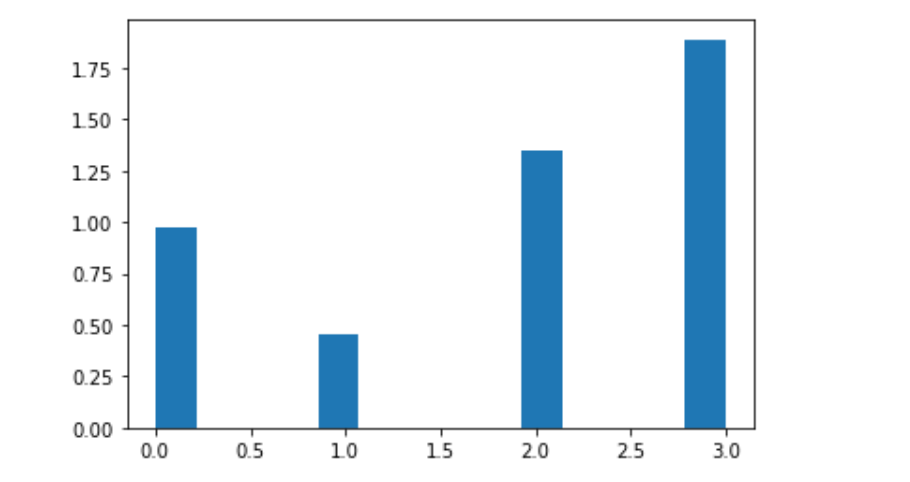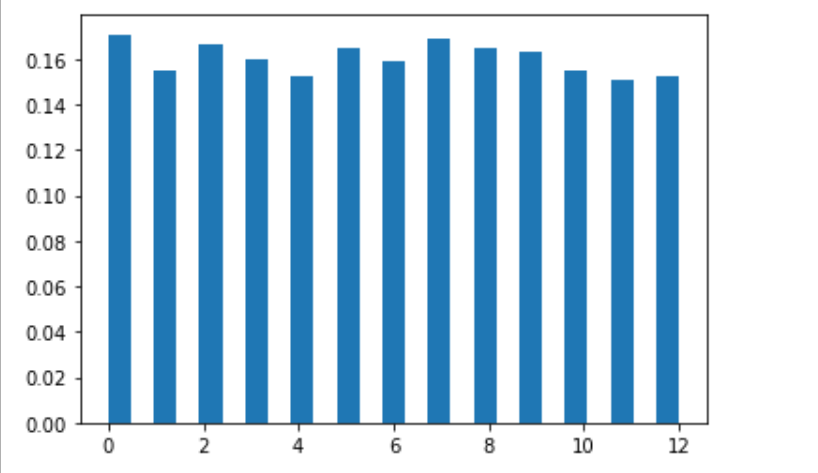### Python Numpy random choice()

Description
NumPy generally stands for Numerical Python and it is a general purpose array processing package.it always provides a high performance multidimensional array object. Numpy is usually for creating an array of n dimensions.

Method Name :numpy.random.choice(a, size=None, replace=True, p=None)
Description :The choice() method, we can get the random samples of one dimensional array and return the random samples of numpy array. The random choice() method always returns a randomly selected element from a non-empty sequence but An empty sequence as an argument raises an IndexError.This function is not accessible directly, so we need to import a random module and then we need to call this function using the random static object.

Parameter Name: a
Description :1-D array of numpy having random samples.

Parameter Name: size
Description :Output shape of random samples of numpy array.

Parameter Name: replace
Description : Whether the sample is with or without replacement.

Parameter Name: p
Description :The probability attach with every sample in array

Return : Return the numpy array of random samples.

The first step would be to give syntax
command:
numpy.random.choice(a, size=None, replace=True, p=None)

Example :
Code :
# import choice import numpy as np import matplotlib.pyplot as plt # Using choice() method gfg = np.random.choice(5, 1000, p =[0.2, 0.1, 0.3, 0.4, 0]) count, bins, ignored = plt.hist(gfg, 14, density = True) plt.show()

Output :Example :
Code :
# import choice import numpy as np import matplotlib.pyplot as plt # Using choice() method gfg = np.random.choice(13, 5000) count, bins, ignored = plt.hist(gfg, 25, density = True) plt.show()

Output :